# TSA Capon Spectrum VI

LabVIEW 2014 Advanced Signal Processing Toolkit Help

Edition Date: June 2014

Part Number: 372656C-01

»View Product InfoDownload Help (Windows Only)

Owning Palette: Correlation and Spectral Analysis VIs

Computes the single-sided power spectral density (PSD) of a univariate time series Xt by using the Capon method. The Capon method uses a nonparametric method based on finite impulse response (FIR) filters to estimate the signal spectrum. Wire data to the Xt input to determine the polymorphic instance to use or manually select the instance.

Example

Use the pull-down menu to select an instance of this VI.

 Select an instance TSA Capon Spectrum (Waveform)TSA Capon Spectrum (Real)TSA Capon Spectrum (Complex)

## TSA Capon Spectrum (Waveform)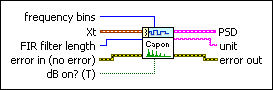frequency bins specifies the number of frequency bins for which this VI computes the single-sided power spectral density PSD. The length of the single-sided PSD is (frequency bins/2+1). The default is 1024.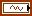Xt specifies the univariate time series.FIR filter length specifies the length, in samples, of the finite impulse response (FIR) filter this VI uses to estimate the PSD. FIR filter length must be less than n–10 where n is the length of Xt.error in describes error conditions that occur before this node runs. This input provides standard error in functionality.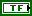dB on? specifies whether this VI returns the PSD in decibels or in a linear scale. If dB on? is TRUE, this VI returns the PSD in decibels. If dB on? is FALSE, this VI returns the PSD in a linear scale. The default is TRUE.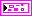PSD returns information about the single-sided power spectral density (PSD).f0 returns the lower boundary, in hertz, of the frequency range.df returns the frequency increment, in hertz.S(f) returns the magnitude of the PSD at each frequency. The value of dB on? determines the unit of measurement for this parameter.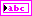unit returns the engineering unit of the PSD. You can specify an engineering unit for a time series by using the TSA Scale to EU VI.error out contains error information. This output provides standard error out functionality.

## TSA Capon Spectrum (Real)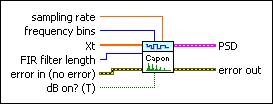sampling rate specifies the sampling rate, in hertz, of the univariate time series Xt. The default is 1.frequency bins specifies the number of frequency bins for which this VI computes the single-sided power spectral density PSD. The length of the single-sided PSD is (frequency bins/2+1). The default is 1024.Xt specifies the univariate time series.FIR filter length specifies the length, in samples, of the finite impulse response (FIR) filter this VI uses to estimate the PSD. FIR filter length must be less than n–10 where n is the length of Xt.error in describes error conditions that occur before this node runs. This input provides standard error in functionality.dB on? specifies whether this VI returns the PSD in decibels or in a linear scale. If dB on? is TRUE, this VI returns the PSD in decibels. If dB on? is FALSE, this VI returns the PSD in a linear scale. The default is TRUE.PSD returns information about the single-sided power spectral density (PSD).f0 returns the lower boundary, in hertz, of the frequency range.df returns the frequency increment, in hertz.S(f) returns the magnitude of the PSD at each frequency. The value of dB on? determines the unit of measurement for this parameter.error out contains error information. This output provides standard error out functionality.

## TSA Capon Spectrum (Complex)sampling rate specifies the sampling rate, in hertz, of the univariate time series Xt. The default is 1.frequency bins specifies the number of frequency bins for which this VI computes the single-sided power spectral density PSD. The length of the single-sided PSD is (frequency bins/2+1). The default is 1024.Xt specifies the univariate time series.FIR filter length specifies the length, in samples, of the finite impulse response (FIR) filter this VI uses to estimate the PSD. FIR filter length must be less than n–10 where n is the length of Xt.error in describes error conditions that occur before this node runs. This input provides standard error in functionality.dB on? specifies whether this VI returns the PSD in decibels or in a linear scale. If dB on? is TRUE, this VI returns the PSD in decibels. If dB on? is FALSE, this VI returns the PSD in a linear scale. The default is TRUE.PSD returns information about the single-sided power spectral density (PSD).f0 returns the lower boundary, in hertz, of the frequency range.df returns the frequency increment, in hertz.S(f) returns the magnitude of the PSD at each frequency. The value of dB on? determines the unit of measurement for this parameter.error out contains error information. This output provides standard error out functionality.

## Example

Refer to the Power Spectral Density Estimation VI in the labview\examples\Time Series Analysis\TSAGettingStarted directory for an example of using the TSA Capon Spectrum VI.